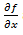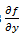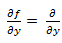Goseeko blog# What are partial differentiation?

## Overview

When we find the area of a triangle then it depends on base and height, hence we can say that area of the triangle is the function of base and height. In other words, when we have a mathematical function having more than one variables, we use partial differentiation.

Symbolically and mathematically the relationship is defined as below

z is called a function of two variables x and y if z has one definite value for every pair of values of x and y.

it is written as

z = f (x, y)

The variable x and y are called independent variables while z is called the dependent variable.

Note- the function z = f (x, y) represents a surface.

## Partial derivatives

First order partial differentiation

Let f(x , y) be a function of two variables. Then the partial derivative of this function with respect to x can be written asand defined as follows:

Now the partial derivative of f with respect to f can be written asand defined as follows:

Note: a. while calculating partial derivatives treat all independent variables, other than the variable with respect to which we are differentiating , as constant.

b. we apply all differentiation rules.

Higher order partial differentiation-

Let f(x , y) be a function of two variables. Then its second-order partial derivatives, third order partial derivatives and so on are referred as higher order partial derivatives.

These are second order four partial derivatives:

b and c are known as mixed partial derivatives.

Similarly we can find the other higher order derivatives.

## Solved examples

Example-1: Calculate for the following function

f(x , y) = 3x³-5y²+2xy-8x+4y-20

Sol. To calculate the variable y as a constant, then differentiate f(x,y) with respect to x by using differentiation rules,

Similarly partial derivative of f(x,y) with respect to y is:

Example-2: Calculate for the following function

f( x, y) = sin(y²x + 5x – 8)

Sol. To calculatethe variable y as a constant, then differentiate f(x,y) with respect to x by using differentiation rules,

Similarly the partial derivative of f(x,y) with respect to y is,[sin(y²x + 5x – 8)]

= cos(y²x + 5x – 8) (y²x + 5x – 8)

= 2xycos(y²x + 5x – 8)

Interested in learning about similar topics? Here are a few hand-picked blogs for you!